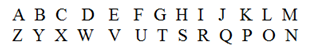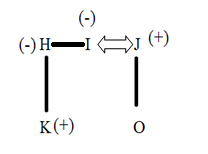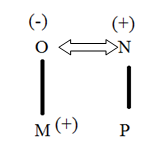# Crack SBI Clerk / IDBI Executive 2018 – Logical Reasoning (Coding Decoding & Inequality) Day-56

Dear Readers, Reasoning Section play a vital role in Banking and all other competitive exams. This year 2018 was started with three wonderful opportunities; Most Awaited SBI Clerk 2018. To enrich your preparation here we have providing new series of Practice Questions on Reasoning Ability – Coding Decoding & Inequality. Candidates those who are going to appear in Bank Exams 2018 can practice these questions daily and make your preparation effective.

Click “Start Quiz” to attend these Questions and view Explanation

Directions (1-5): Study the given information carefully and answer the given questions.

“Seven Two Four” is written as “[email protected]  [email protected]  U10*”

“Nine Three Eight” is written as “M10* [email protected]  [email protected]

“Six Ten Forty” is written as “[email protected]  [email protected]  [email protected]

1) What is the code for “Aspirant”?

a) A4*

b) Z4*

c) Z8*

d) Y7*

e) None of these

2) What is the Code “Good person”?

a) T10* [email protected]

b) T10* K9*

d) T9* K10*

e) None of these

3) What is the Code for “Twenty Eight”?

a) G9* [email protected]

c) [email protected] V12*

d) G12* [email protected]

e) None of these

4) What is the Code for “Logical Thinking”?

a) [email protected] G8*

b) O10* G8*

d) O10* [email protected]

e) None of these

5) What Could ‘[email protected]’ Stand for?

a) Mistake

b) Make

c) Monkey

d) Move

e) None of these

Directions (6- 8): Study the given information carefully and answer the given questions.

6) In which of the following expressions will the expression ‘H > J’ be definitely true?

a) G < H ≥ I = J

b) H > G ≥ I = J

c) J = I ≥ G > H

d) H ≥ G > I < J

e) None of these

7) Which of the following expressions will be true if the expression ‘P ≥ Q > R ≥ S’ is definitely true?

a) P ≤ S

b) Q = S

c) P > S

d) R ≥ P

e) None is true

8) Which of the following expressions will be true if the expression ‘P ≥ R < T = Q’ is definitely true?

a) Q < P

b) Q ≥ T

c) R < Q

d) T = P

e) None is true

Directions (9 – 10): Study the following carefully and answer the given questions.

A @ B Means A is wife of B

A* B means A is sister of B

A % B means A is son of B

A#B means A is Mother of B

A\$B means A is father of B

9) How is J is related to K in the given Expression  K %H*[email protected]\$O?

a) Son

b) Father

c) Brother

d) Uncle

e) None of these

10) Which of the following expressions represents M is Brother of P?

b) M%O*[email protected]

c) M%[email protected]\$P

d) [email protected]%N#P

e) None of these

Seven

1) S = H2) Seven = 5

5*5*5 = 125

1+2+5= 8

3) Odd = @

Even = *

3. Answer a) G9* [email protected]
4. Answer a) [email protected] G8*

Directions (6- 8):

6. Answer b) H > G ≥ I = J

7. Answer c) P > S

8. Answer c) R < Q

Directions (9-10):